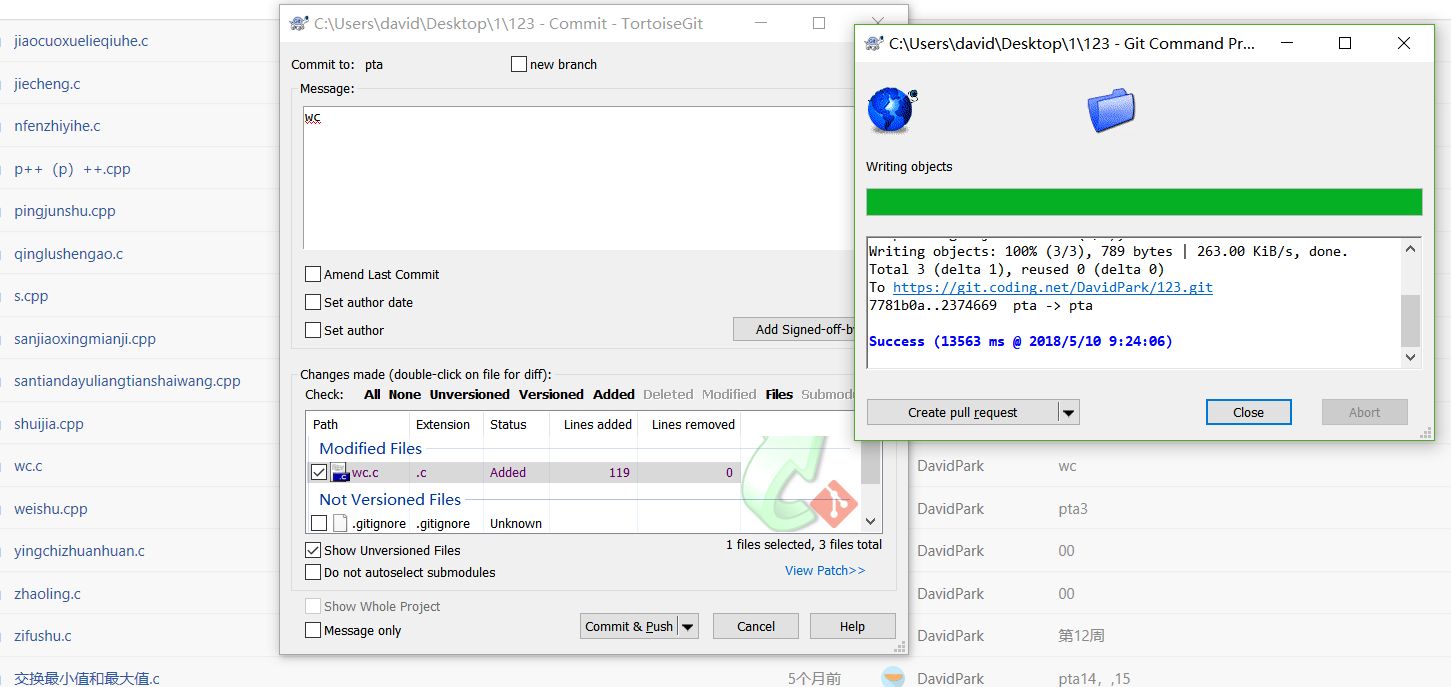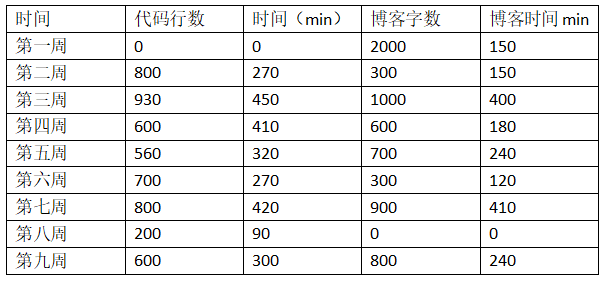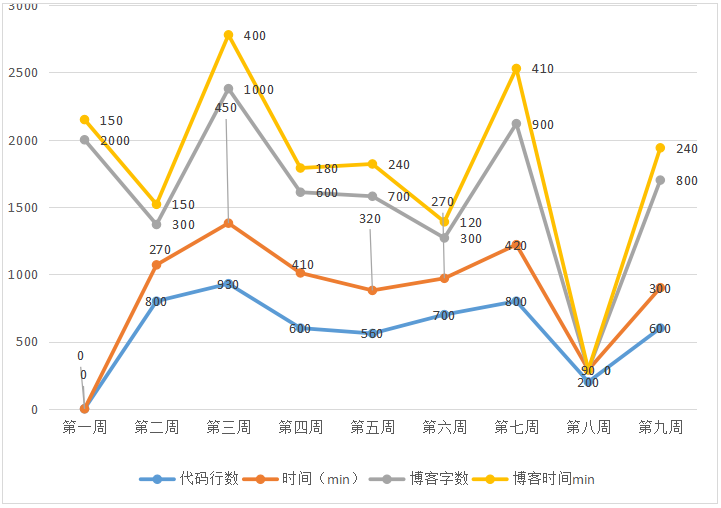# 一，要求一：

## 2).实验代码：

	#include <stdio.h>
#include <string.h>

int main( int argc,char *argv[] )
{
int lines;
int numbers;
int words;
while( argv )
{
if( strcmp(argv,"-l") == 0)
{
lines = Line(argv);
printf( "%sµÄÐÐÊý = %d\n",argv,lines );
}
if( strcmp(argv,"-c") == 0)
{
numbers = char(argv) - 1;
printf( "%sµÄ×Ö·ûÊý = %d\n",argv,numbers );
}
if( strcmp(argv,"-w") == 0)
{
words = wor(argv) - 1;
printf( "%sµÄ´ÊµÄÊýÄ¿ = %d\n",argv,words );
}
break;
}
}

int Line( char *file_name )
{
int lines;
char ch;
FILE *fp;
fp = fopen( file_name,"r" );
if( fp == NULL )
{
printf( "File Open Error!\n" );
return 0;
}
ch = fgetc(fp);
lines = 0;
while( ch != EOF )
{
if( ch == '\n' )
{
lines = lines + 1;
}
ch = fgetc(fp);
}
if( fclose(fp) )
{
printf( "Can Not Close The File!\n" );
return 0;
}
return lines;
}
int char( char *file_name )
{
int num;
char ch;
FILE *fp;
fp = fopen( file_name,"r" );
if( fp == NULL )
{
printf( "File Open Error!\n" );
return 0;
}
ch = fgetc(fp);
num = 0;
while( ch != EOF )
{
num = num + 1;
ch = fgetc(fp);
}
if( fclose(fp) )
{
printf( "Can Not Close The File!\n" );
return 0;
}
return num;
}
int word( char *file_name )
{
int word,f;
char ch;
FILE *fp;
fp = fopen( file_name,"r" );
if( fp == NULL )
{
printf( "File Open Error!\n" );
return 0;
}
ch = fgetc(fp);
word = 0;
f = 0;
while( ch != EOF )
{
if(((ch >= 'a')&&(ch <= 'z'))||((ch >= 'A')&&(ch <= 'Z')))
{
f = 0;
}else
{
if(f == 0)
{
word = word + 1;
f = 1;
}
}
ch = fgetc(fp);
}
if( fclose(fp) )
{
printf( "Can Not Close The File!\n" );
return 0;
}
return word;
}


# 二，要求二：

## 1.git：

Git地址## 3.用表格和折线图呈现你本学期的代码行数和所用时间、博客字数和所用时间:## 6.对教师说的，建议、抱怨、希望…

posted @ 2018-05-04 22:59  DavidPark  阅读(225)  评论(9编辑  收藏  举报